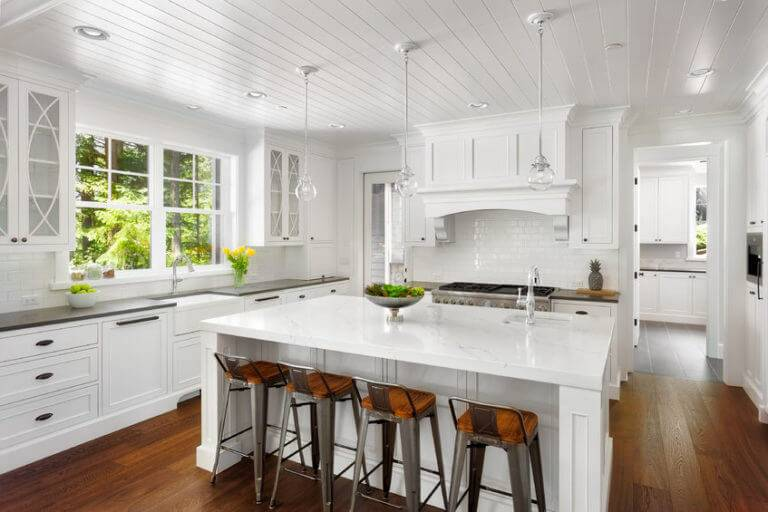# Calculator

## How to calculate square footage?Accurately calculating square footage is a major step in any home improvement project. This measurement is necessary to purchase the right amount of materials for your project and or to define the size of a specific area or space. Calculating square footage requires careful measurements and multiplication. Feel free to use this as a guide to measuring the square feet prior to diving deep in your next project.

### Step 1: Measure

Measure all the sides of the area. More specifically, measure the length and width. Double-check the accuracy by measuring at least two or three times. An important tip is to ensure all measurements are in the same unit. For example, take note of whether you are measuring in inches, centimetres, feet, yards, etc. All measurements must be in feet, considering square footage is expressed in that particular unit. Those units used need to be converted into feet before moving on to the next steps in the calculation. For example: Length of 72 inches is equivalent to 6 feet Width of 24 inches is equivalent to 2 feet To convert any unit into feet, click here.

Once again, all measurement units must be in feet in order to correctly calculate square footage.

### Step 2: Apply the Square Footage Formula

The square footage formula is the same formula used to calculate the area. Spaces shaped like squares or rectangles, multiply the length measurement by the width. In better words, length multiplied by the width = square footage. For example:

Length of 6 feet

Width of 2 feet

Length X width = square footage | 6 X 2 = 12 Square Feet

For areas, not square or rectangle shape will require a different formula.

Here is a list of all formulas to calculate the square footage of any shaped space.

In cases where spaces may require odd dimensions, meaning the area does not have a defined shape, break up the area in measurable rectangles and squares. Calculate the area for each section using the formula length times width, then add the areas of each section together.

This is helpful to measure T-shaped, L-shaped, or any other odd-shaped space.

### Step 3: Adjust for Calculator Errors

After you have accurately calculated the square footage of a space, adjust for error. This is especially important for those planning to purchase materials based on the measurements. It is common for mistakes to occur during such projects. Plan in advance for these mistakes, then prepare by ordering additional materials.

A fair measurement of error is 5 to 10% of the calculated square footage. For example:

Measured square footage = 12 square feet

12 square feet X 5% = .6 square feet

12 square feet X 10% = 1.2 square feet

.6 square feet + 1.2 square feet = 1.8 square feet

Most materials purchased are ordered in whole amounts. In cases where calculations result in decimals, round to the nearest whole number. For example, 1.8 square feet is rounded to 2 square feet.

For questions about calculating the square footage, our experts are ready to help. Contact our team at Stone Spirit today.